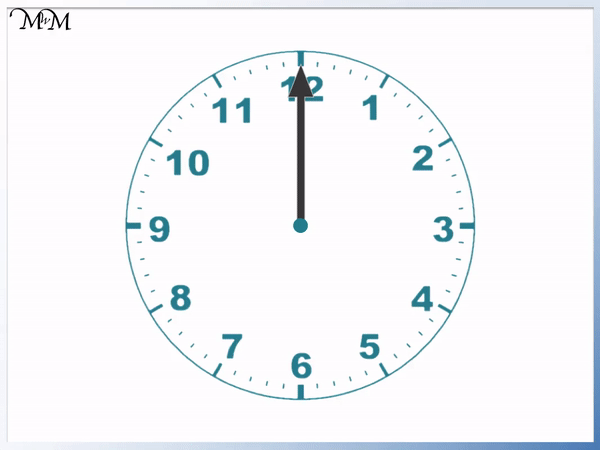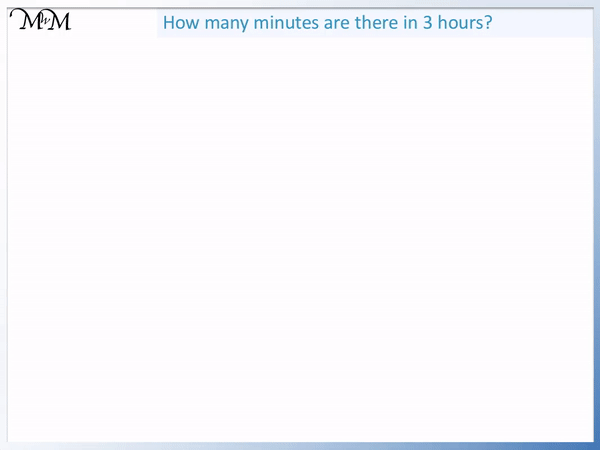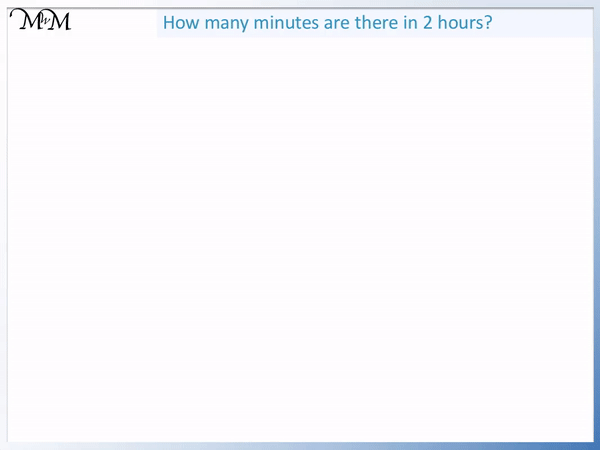# Minutes in an Hour and Hours in a Day

Minutes in an Hour and Hours in a Day• Each of the smallest lines on a clock face is 1 minute.
• An hour will have passed when the largest hand (the minute hand) completes a full turn.
• We will count how many minutes pass until the minute hand returns back to the top (pointing at the 12).
• As the minute hand moves to point at the 1, five minutes have passed.
• As the minute hand moves from one number on the clock face to the next, 5 more minutes pass.
• We can skip count by 5 to find the total number of minutes in an hour.
• We have 5, 10, 15, 20, 25, 30, 35, 40, 45, 50, 55 and 60
• There are 60 minutes in an hour and 30 minutes in half an hour.

There are 5 minutes between each number on the clock face.

There are 60 minutes in an hour and 30 minutes in half an hour.• A full day contains 24 hours.
• A day is split into 12 hours in the morning and 12 hours in the afternoon.
• We write ‘am’ for times in the morning and ‘pm’ for times in the afternoon.
• The day begins at twelve o’clock am, which is in the morning (it will be dark outside).
• Once 12 hours have passed we reach twelve o’clock pm, which is half way through the full day (it will be light outside now).
• This is midday (or noon) and these next 12 hours will have ‘pm’ written to show that it is afternoon.
• 12 hours pass until noon (half way through the day) and then 12 more hours pass until the end of the day
• The hour hand points at each number twice in a day, once for ‘am’ and once for ‘pm’

There are 24 hours in a day.

12 hours are in the morning and 12 hours are in the afternoon.• There are 60 minutes in every hour.
• To find the number of minutes in 3 hours, we multiply 60 by 3.
• To multiply by 60, multiply first by 6 and then multiply the answer by 10.
• 6 x 3 = 18 and so 60 x 3 = 180.
• There are 180 minutes in 3 hours.#### Teaching the Number of Hours in a Day# The Number of Minutes in an Hour and The Number of Hours in a Day

There are 60 minutes in an hour and 24 hours in a day.

To work out the number of minutes in a time given in hours, multiply the number of hours by 60.

To convert a time in days to a time in hours, multiply the time in days by 24.

We will look at some examples of how to convert hours to minutes and days to hours further in this lesson.

## Converting Hours to Minutes

The largest hand on an analogue clock is called the minute hand. The smallest line on the scale of a clock face is worth one minute. It takes one minute for the minute hand to move between each of the smallest lines shown.

The smaller hand is the hour hand.

The day starts with both hands on the clock face pointing upwards to the 12.

We can count how many minutes there are until the minute hand reaches the number ‘1’.

The number ‘1’ is 5 lines away from the ’12. There are five minutes between the ’12’ and the ‘1’ on the clock.

We can count the minutes as the hand moves: 1, 2, 3, 4, 5.

Each number that is written on the clock face is separated by 5 minutes.Each written number on the clock face has a slightly larger line. Every time the minute hand reaches a new number, another five minutes have passed.

This means that we can skip count in fives instead of counting every single minute mark shown.

We can multiply the number written on the clock face by 5 to see how many minutes have passed.

2 x 5 = 10 and so, when the minute hand points at the 2, ten minutes have passed.

3 x 5 = 15 and so, when the minute hand points at the 3, fifteen minutes have passed.

Continuing to count in fives we have: 20, 25, 30, 35, 40, 45, 50, 55 and finally we reach 60 once the minute hand returns to the top.

It has taken 60 minutes for the minute hand to rotate fully around the clock to return to its original position.

There are 60 minutes in an hour. We can see that 12 x 5 = 60. The minute hand points at ’12’.

No matter where the minute hand starts, it will take 60 minutes to return to the same starting position, which is one hour later.

Once we know that there are 60 minutes in an hour, it is easy to find how many minutes there are in two hours.

We could continue to count on from 60 minutes in fives until the minute hand has rotated fully to make another hour.We can see that another 60 minutes has passed in the second hour.

The first hour was 60 minutes and the second hour was another 60 minutes.

60 + 60 = 120

In total two hours is 120 minutes.

However, when teaching converting hours to minutes it is easiest to multiply the number of hours by 60.

2 hours is 2 lots of 60 minutes and so, the total time is 60 x 2.Multiplying by 60 may seem a little daunting for a child because it is a much larger number than those in the times tables.

Fortunately, multiplying by 60 is a relatively rare calculation in primary school mathematics, predominantly featuring in this time topic.

The easiest way to multiply by 60 is to multiply by 6 using times tables and then to multiply by 10 afterwards.

We can use this trick to find the number of minutes in 3 hours.

We need to calculate 60 x 3.

We can use the fact that 6 x 3 = 18.

60 x 3 = 180, which is ten times larger.There are 180 minutes in three hours.

Similarly we can work out the number of minutes in four hours by multiplying 60 by 4.

6 x 4 = 24 and so, 60 x 4 = 240.There are 240 minutes in 4 hours.

Since there are 60 minutes in a whole hour, we can halve this amount to find the minutes in every half hour.

Half of 60 is 30 and so, there are 30 minutes in half an hour.

We can halve this again to find one quarter. There are 15 minutes in a quarter of an hour.

For example: How many minutes are in 2 and a half hours?

We can work out the number of minutes in 2 hours and then add the half hour separately at the end.

2 x 60 = 120 and so, there are 120 minutes in two hours.

The extra half an hour is 30 minutes.

12 + 3 = 5 and so, 120 + 30 = 150.

There are 150 minutes in 2 and a half hours.

## Converting Days to Hours

A new day begins at twelve o’clock in the morning, which can be written as 0:00 am.

This means that we are at the start of the day because zero hours have passed.

Noon is the middle of the day at 12:00 pm. It is also called midday.

Each day there are 12 hours before noon and 12 hours after noon.

For times that are before noon, we write ‘am’ after the time. The ‘am’ is a short way of telling us that this time is in the morning.

For times that are after noon we write ‘pm’ after the time. This is a short way to tell us that the time is after noon.‘am’ is short for ‘Ante-Meridiem’, which is Latin for ‘before noon’.

‘pm’ is short for ‘Post-Meridiem’, which is Latin for ‘after noon’.

We can see that the clock only has 12 numbers and that the day has 24 hours. Each number on the clock face will be pointed at twice, once in the morning and once in the afternoon. To tell the two times apart we need to write ‘am’ or ‘pm’ after the times.

In this example we will calculate how many hours there are in 2 days.

We use the fact that there are 24 hours in one day.

To find the number of hours in 2 days, we multiply 24 by 2.The easiest way to multiply 24 by two is to double each digit separately, since both digits are less than 5.

2 x 2 = 4 and 4 x 2 = 8.

Therefore 24 x 2 = 48.

There are 48 hours in two days.

We can find the number of hours in any given amount of days by multiplying 24 by the number of days.

Similarly we can find the number of hours in half a day.

If there are 24 hours in a whole day, we can halve this amount to see that there are 12 hours in half a day.We already knew that there were 12 hours in the morning and 12 hours in the afternoon. 12 hours in each half of the day.

Using the fact that there are 24 hours in a whole day and 12 hours in half a day, it is possible to work out other combinations for converting days into hours.

For example, how many hours are there in a day and a half?

To calculate this, it is easiest to work out the days and the half days separately and add them at the end.There are 24 hours in one day and 12 hours in half a day.

24 + 12 = 36 and so, there are 36 hours in a day and a half.

When the calculations involve larger and larger numbers, it can be best to teach this topic by encouraging written methods of addition or multiplication.Now try our lesson on Telling Time (Analogue Clock): Half Past where we learn how to read half past the clock.error: Content is protected !!